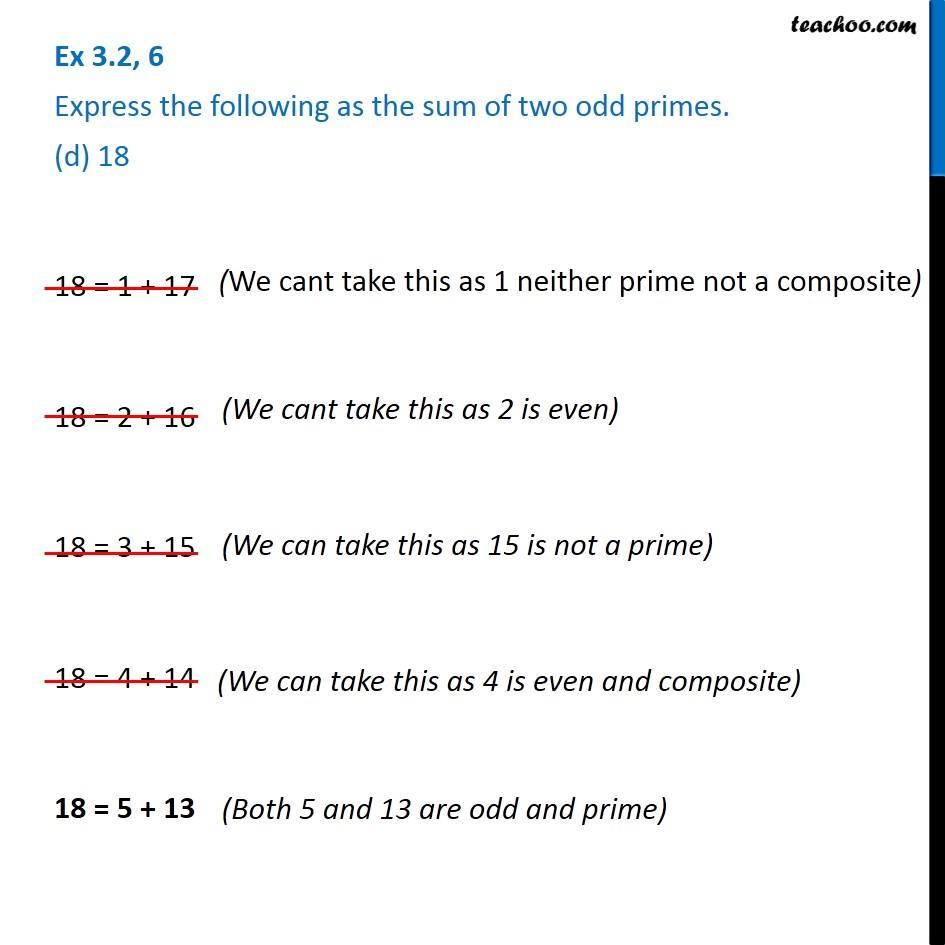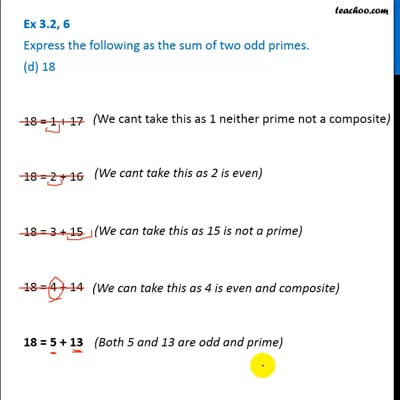Ex 3.2

Chapter 3 Class 6 Playing with Numbers
Serial order wiseThis video is only available for Teachoo black users

Introducing your new favourite teacher - Teachoo Black, at only ₹83 per month

### Transcript

Ex 3.2, 6 Express the following as the sum of two odd primes. (d) 18 18 = 1 + 17 (We cant take this as 1 neither prime not a composite) 18 = 2 + 16 (We cant take this as 2 is even) 18 = 3 + 15 (We can take this as 15 is not a prime) 18 = 4 + 14 (We can take this as 4 is even and composite) 18 = 5 + 13 (Both 5 and 13 are odd and prime)